Next: Inductance Up: AC Circuit Elements Previous: AC Circuit Elements

## Capacitance

The fundamental property of a capacitor is that it can store charge and hence electric field energy. The capacitance C between two appropriate surfaces is defined by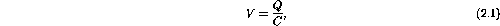where V is the potential difference between the surfaces and Q is the magnitude of the charge distributed on either surface.

In terms of current, I = dQ/dt implies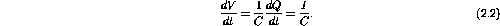In electronics we take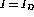(displacement current). In other words, the current flowing from or to the capacitor is taken to be equal to the displacement current through the capacitor. You should be able to show that capacitors add linearly when placed in parallel.

There are four principle functions of a capacitor in a circuit.

1. Since Q andcan be stored a capacitor can be used as a (non-ideal) source of I and V.
2. Since a capacitor passes AC current but not DC current it can be used to connect parts of a circuit that must operate at different DC voltage levels.
3. A capacitor and resistor in series will limit current and hence smooth sharp edges in voltage signals.
4. Charging or discharging a capacitor with a constant current results in the capacitor having a voltage signal with a constant slope, ie. dV/dt = I/C = constant if I is a constant.

Some capacitors (electrolytic) are asymmetric devices with a polarity that must be hooked-up in a definite way. You will learn this in the lab. The SI unit for capacitance is farad (F). The capacitance in a circuit is typically measured inF or pF. Non-ideal circuits will have stray capacitance, leakage currents and inductive coupling at high frequency. Although important in real circuit design we will slip over these nasties at this point.

Capacitors can be obtained in various tolerance ratings from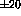% to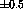%. Because of dimensional changes, capacitors have a high temperature dependence of capacitance. A capacitor does not hold a charge indefinitely because the dielectric is never a perfect insulator. Capacitors are rated for leakage, the conduction through the dielectric, by the leakage resistance-capacitance product in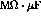. High temperature increases leakage.Next: Inductance Up: AC Circuit Elements Previous: AC Circuit Elements

Doug Gingrich
Tue Jul 13 16:55:15 EDT 1999